Courses

# Ex 14.1 NCERT Solutions- Statistics Class 10 Notes | EduRev

## Class 10 : Ex 14.1 NCERT Solutions- Statistics Class 10 Notes | EduRev

The document Ex 14.1 NCERT Solutions- Statistics Class 10 Notes | EduRev is a part of the Class 10 Course Class 10 Mathematics by VP Classes.
All you need of Class 10 at this link: Class 10

NCERT TEXTBOOK QUESTIONS SOLVED
EXERCISE 14.1

Page No. 270
Q 1. A survey was conducted by a group of students as a part of their environment awareness programme, in which they collected the following data regarding the number of plants in 20 houses in a locality. Find the mean number of plants per house.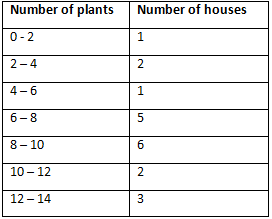Which method did you use for finding the mean, and why?
Sol. We can calculate the mean as: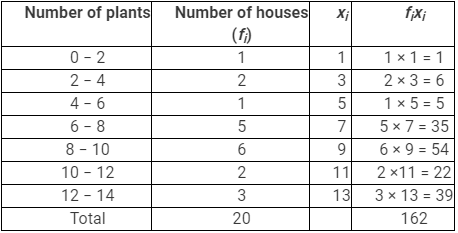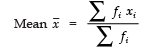⇒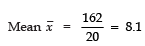Thus, mean number of plants per house is 8.1.
Since, values of xi and fi are small
∴ We have used the direct method.

Q 2. Consider the following distribution of daily wages of 50 workers of a factory.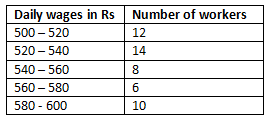Find the mean daily wages of the workers of the factory by using an appropriate method.
Sol.
To find the class mark for each interval, the following relation is used.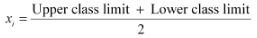Class size (h) of this data = 20
taking 550 as assured mean (a), di, uj and fiuj can be calculated as follows.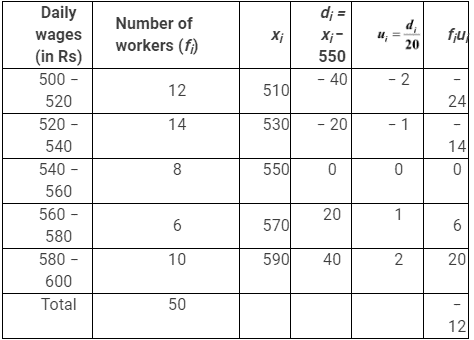From the table, it can be observed that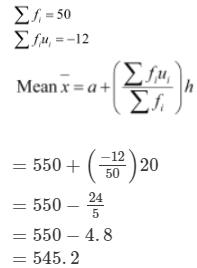Therefore, the mean daily wage of the workers of the factory is Rs 545.20

Q 3. The following distribution shows the daily pocket allowance of children of a locality. The mean pocket allowance is Rs 18. Find the missing frequency f.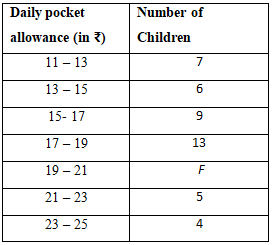Sol. Let the assumed mean, a = 16
∵ Class interval h = 2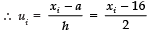Now, we have the following table: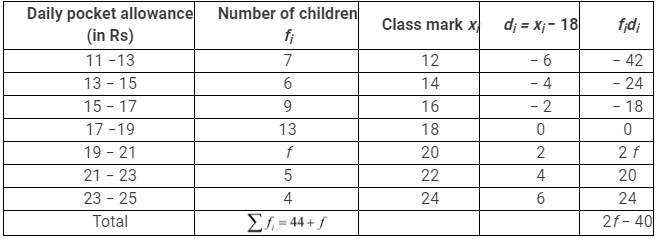Since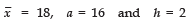∴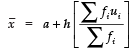⇒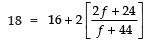⇒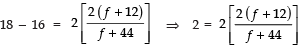⇒ f + 44 = 2 (f + 12) = 2f + 24
⇒− f = −44 + 24 = −20
⇒ f = 20
Thus, the missing frequency is 20.

Page No. 271
Q 4. Thirty women were examined in a hospital by a doctor and the number of heart beats per minute were recorded and summarised as follows. Find the mean heart beats per minute for these women, choosing a suitable method.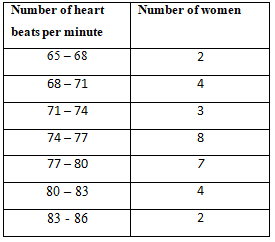Sol. Let the assumed mean a = 75.5
∴ Class interval h = 3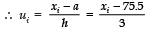Now, we have the following table: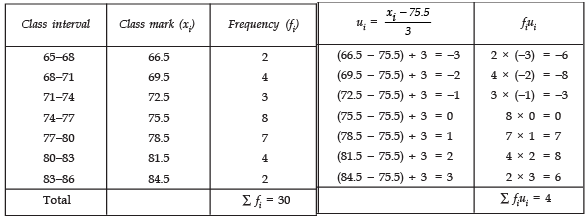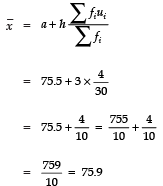Thus, the mean heart beat per minute is 75.9.

Q 5. In a retail market, fruit vendors were selling mangoes kept in packing boxes. These boxes contained varying number of mangoes. The following was the distribution of mangoes according to the number of boxes.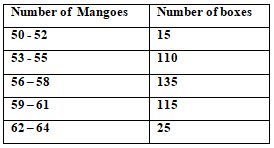Find the mean number of mangoes kept in a packing box. Which method of finding the mean did you choose ?
Sol. Let the assumed mean a = 60
Here, Class interval h = 3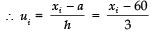Now, we have the following table: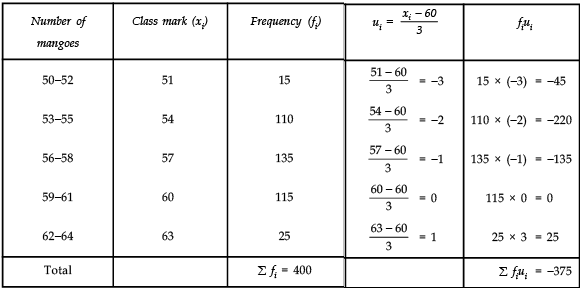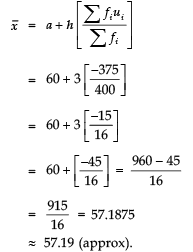Thus, the average number of mangoes per box = 57.19.

Q 6. The table below shows the daily expenditure on food of 25 households in a locality.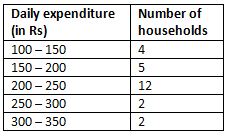Find the mean daily expenditure on food by a suitable method.
Sol. Let the assumed mean, a = 225
And class interval h = 50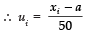∴ We have the following table: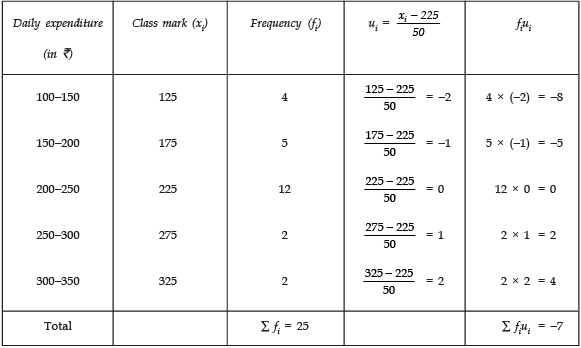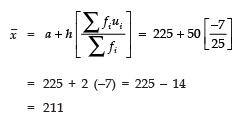Thus, the mean daily expenditure of food is Rs 211.

Q 7. To find out the concentration of SO2 in the air (in parts per million, i.e., ppm), the data was collected for 30 localities in a certain city and is presented below: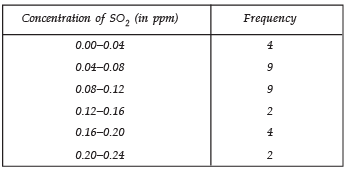Find the mean concentration of SO2 in the air.
Sol. Let the assumed mean a = 0.14
Here, class interval h = 0.04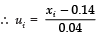∴ We have the following table: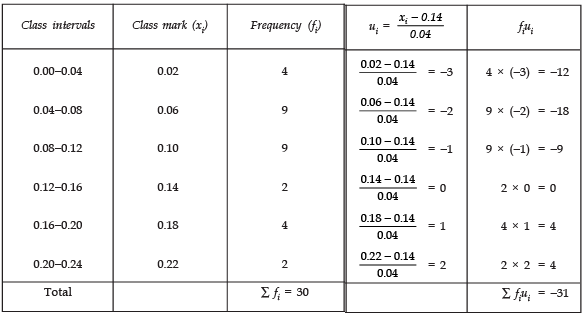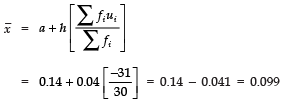Thus, mean concentration of SO2 in air is 0.099 ppm.

Page No. 272
Q 8. A class teacher has the following absentee record of 40 students of a class for the whole term. Find the mean number of days a student was absent.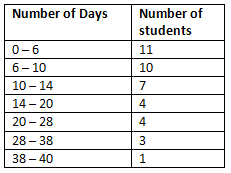Sol. Using the direct method, we have the following table: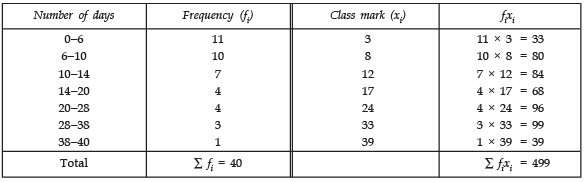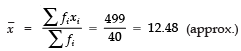Thus, mean number of days a student remained absent = 12.48. (Approx.)

Q 9. The following table gives the literacy rate (in percentage) of 35 cities. Find the mean literacy rate.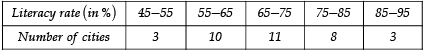Sol. Let  assumed mean a =70
∴ Class interval h =10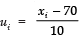Now, we have the following table: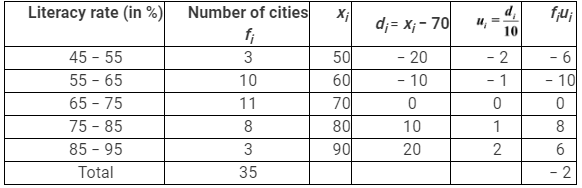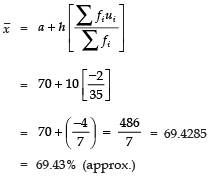Thus, the mean literacy rate is 69.43%.

Offer running on EduRev: Apply code STAYHOME200 to get INR 200 off on our premium plan EduRev Infinity!

132 docs

,

,

,

,

,

,

,

,

,

,

,

,

,

,

,

,

,

,

,

,

,

;